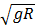# A satellite is revolving in a circular orbit at a height h from the earth surface. Such that h<<R where R is the radius of the earth. Assuming that the effect of earth’s atmosphere can be neglected the minimum increase in the speed required so that the satellite could escaped from the gravitational field of earth is : (a)((b)(c)(d)## Question ID - 50305 :- A satellite is revolving in a circular orbit at a height h from the earth surface. Such that h<<R where R is the radius of the earth. Assuming that the effect of earth’s atmosphere can be neglected the minimum increase in the speed required so that the satellite could escaped from the gravitational field of earth is : (a)((b)(c)(d)3537

V0=Ve=v=ve−v0=(Next Question :
 In an experiment electrons are accelerated, from rest, by applying a voltage of 500 V. calculate the radius of the path if a magnetic field 100 mT is then applied. [charge of the electron=1.6×10−19C Mass of the electron =9.1×10−31kg] (a) 7.5×10−4m (b) 7.5×10−3 m (c) 7.5 m (d) 7.5×10−2 m